NEW!  CBSE Papers PDF: Class-X, Class-XII

# (Download) CBSE Class-12 Sample Paper And Marking Scheme 2015-16 :  Physics

Disclaimer: This website is not at associated with CBSE, For official website of CBSE visit - www.cbse.nic.in

#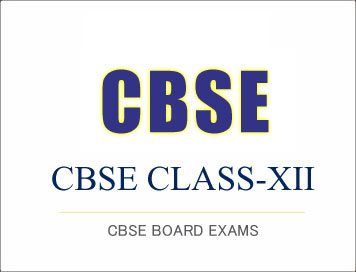## (Download) CBSE Class-12 Sample Paper And Marking Scheme 2015-16 :  Physics

Sample Question Paper

Physics

Class XII

Time Allowed: 3 Hours    Maximum Marks: 70

General Instructions

1.  All questions are compulsory. There are 26 questions in all.

2.  This question paper has five sections: Section A, Section B, Section C, Section D and Section E.

3.  Section A contains five questions of one mark each, Section B contains five questions of two marks each,  Section C contains twelve questions of three marks each, Section D contains one value based question of  four marks and Section E contains three questions of five marks each.
4.  There is no overall choice. However, an internal choice has been provided in one question of  two marks,  one question  of three marks and  all  the three questions of five marks weightage.  You have to attempt only one of the choices in such questions.
5.  You may use the following values of physical constants wherever necessary.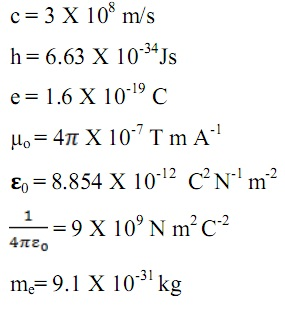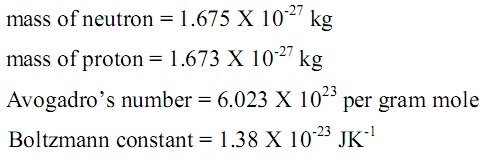Section A

1.  Figure shows a point charge +Q, located at a distance R/2 from the centre of a spherical metal shell. Draw the electric field lines for the given system. (1)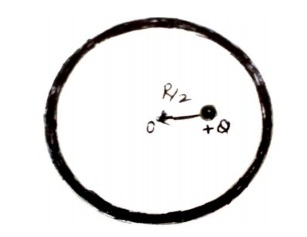2.  Give  an  example  of  a  material  each  for  which  temperature  coefficient  of resistivity is (i) positive, (ii) negative.    (1)

3.  A device ‘X’ is connected to an a.c. source V = Vo  sin ωt. The variation of voltage, current and power in  one complete cycle is shown in the following figure.    (1)

(i) Which curve shows power consumption over a full cycle?
(ii) Identify the device ‘X’.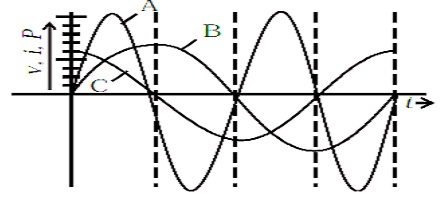4.  An object is placed in front of convex lens made of glass. How does the image distance vary if the refractive index of the medium is increased in such a way that still it remains less than the glass?    (1)

5.  Name the network within an institution, made by connecting all or some of their computers.    (1)

Section B

6.  Two cells of E.M.F. 10 V and 2 V and internal resistances 10 Ω and 5 Ω respectively, are connected in  parallel as shown. Find the effective voltage across R.    (2)

7.  Figure  shows  a  system  of  two  polarizing  sheets  in  the  path  of  initially unpolarized light. The polarizing direction of first sheet is parallel to x-axis and that of second sheet is 60o  clockwise from x-axis.  Calculate what fraction of intensity of light emerges from the system.    (2)